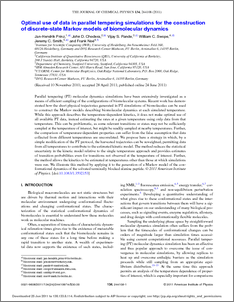Repository: Freie Universität Berlin, Math Department

# Optimal use of data in parallel tempering simulations for the construction of discrete-state Markov models of biomolecular dynamics

Prinz, J.-H. and Chodera, J. D. and Pande, V. S. and Swope, W. D. and Smith, J. C. and Noé, F. (2011) Optimal use of data in parallel tempering simulations for the construction of discrete-state Markov models of biomolecular dynamics. J. Chem. Phys., 134 (24). p. 244108.Preview

1MB

Official URL: http://dx.doi.org/10.1063/1.3592153

## Abstract

Parallel tempering (PT) molecular dynamics simulations have been extensively investigated as a means of efficient sampling of the configurations of biomolecular systems. Recent work has demonstrated how the short physical trajectories generated in PT simulations of biomolecules can be used to construct the Markov models describing biomolecular dynamics at each simulated temperature. While this approach describes the temperature-dependent kinetics, it does not make optimal use of all available PT data, instead estimating the rates at a given temperature using only data from that temperature. This can be problematic, as some relevant transitions or states may not be sufficiently sampled at the temperature of interest, but might be readily sampled at nearby temperatures. Further, the comparison of temperature-dependent properties can suffer from the false assumption that data collected from different temperatures are uncorrelated. We propose here a strategy in which, by a simple modification of the PT protocol, the harvested trajectories can be reweighted, permitting data from all temperatures to contribute to the estimated kinetic model. The method reduces the statistical uncertainty in the kinetic model relative to the single temperature approach and provides estimates of transition probabilities even for transitions not observed at the temperature of interest. Further, the method allows the kinetics to be estimated at temperatures other than those at which simulations were run. We illustrate this method by applying it to the generation of a Markov model of the conformational dynamics of the solvated terminally blocked alanine peptide.

Item Type: Article Physical Sciences Department of Mathematics and Computer Science > Institute of Mathematics > Comp. Molecular BiologyDepartment of Mathematics and Computer Science > Institute of Mathematics > BioComputing Group 993 BioComp Admin 17 Nov 2010 15:41 03 Mar 2017 14:40

Repository Staff Only: item control page